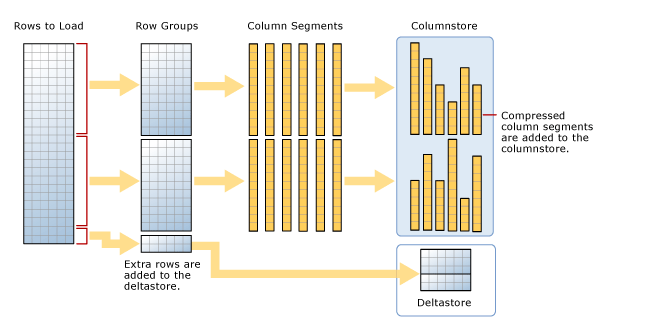In today’s post, we will talk about the structure of the Columnstore indexes, as well as the way in which it works internally to facilitate its understanding.

## 1.- Columnstore.

Let’s refresh the definition of column store. Column store indexes are the standard for storing and querying big sized fact tables. As the name implies, this index is based on columns and the processing of queries reaches a profit of 1000% compared to storage based on rows. The logical structure of a column store is a table with rows and columns, but it is physically stored in a column format.

If we focus on the internal storage of columnstore indexes, we should know that each group of rows will be at least 102,400 rows and 1,048,576 rows at the most. In this way, if we have a table with 2,100,000 records and create an index based on columns on it, it will be stored in 2 groups of rows of 1,048,576 each and there will be 2,848 rows, which are not enough to belong to another group of rows. These leftover rows are inserted into the delta store.

## 2.- What is the Delta Store?

Well, to understand what the delta store is, let’s focuson the previous example.

Having 2 groups of rows with 1,048,576 each, we have 2,848 rows that are not enough to belong to a new group of rows, therefore, these rows are stored in the delta row group, which is no more than an index of type B-tree that is added to the columnstore index.

In the case of massive loads of less than 102,400 rows, these will go directly to the delta store.

There is a “problem” when you have delta stores open, the data is not compressed and this slows down searches on the columnstore index. Therefore, it is convenient to always close the delta store.

There are two ways to close the delta row groups:

• Perform a rebuild of the columnstore index. When the index is reconstructed, the groups of delta rows are closed and will present a completely “segmented” table. It could take a long time, depending on the partitioning and the use we are giving it. An example of rebuild of a columnstore index would be the following:
• ALTER INDEX [Index_Name] ON [dbo].[Table] REBUILD PARTITION = ALL WITH (DATA_COMPRESSION = COLUMNSTORE);
• ALTER INDEX [Index_Name] ON [dbo].[Table] REORGANIZE WITH (COMPRESS_ALL_ROW_GROUPS = ON);
• Perform a massive insertion of rows. If a massive insertion of rows is carried out and they reach the maximum number of rows in the row group (1,048,576), they will be compressed and taken to the columnstore index using the Tuple-Mover process that we will see next.

## 3.- Tuple-Mover

This process checks the closed groups of about 1 million rows that are ready to be compressed and added to the columnstore index.

To understand the above process, we will rely on the following image:As we can see, there are a total number of rows that are going to be loaded, putting the previous example, will be 2,100,000 rows.

These rows will be divided into 2 row groups of 1,048,576 that will be compressed and will be part of the columnstore index, therefore, there will be 2,848 rows that will be stored in the delta store when not reaching the minimum of 102,400 rows to start a new row group.

If we make an insertion of rows, these will be added to the rows in the delta store and when starting the tuple-move process, if it reaches a million rows, they will be compressed and taken to the columnstore index.

Tip: In some version of SQL Server, the tuple-move does not work correctly when the table is being locked by another process such as a Select. Therefore, it is recommended that a rebuild of the index be done manually each time a massive row insertion is done.

## 4.- Non clustered columnstore index

A non-clustered column store index and a clustered column store index work the same way. The difference is that a non-clustered index is a secondary index created in a row store table, but a clustered column store index is the main storage of the entire table.

When performing a search, it can be done on the ungrouped index of the table, which has part of the table in its entirety, but this will really be done on the clustered index of column storage. In new entries we will emphasize columnstore rates for real-time operational analysis.

I hope it has been helpful!